Courses

# Test: Flow Through Pipes - 2

## 10 Questions MCQ Test Topicwise Question Bank for Civil Engineering | Test: Flow Through Pipes - 2

Description
This mock test of Test: Flow Through Pipes - 2 for Civil Engineering (CE) helps you for every Civil Engineering (CE) entrance exam. This contains 10 Multiple Choice Questions for Civil Engineering (CE) Test: Flow Through Pipes - 2 (mcq) to study with solutions a complete question bank. The solved questions answers in this Test: Flow Through Pipes - 2 quiz give you a good mix of easy questions and tough questions. Civil Engineering (CE) students definitely take this Test: Flow Through Pipes - 2 exercise for a better result in the exam. You can find other Test: Flow Through Pipes - 2 extra questions, long questions & short questions for Civil Engineering (CE) on EduRev as well by searching above.
QUESTION: 1

### Two reservoirs at different levels are connected by two parallel pipes of diameter '2d' and 'd'. The ratio of the flows in the two pipes (larger: smaller) is

Solution: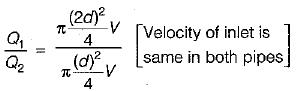⇒ Q1/Q2 = 4/1

QUESTION: 2

### A 15 cm diameter pipe carries a flow of 70 L/s of an oil (RD = 0.75). At a section 12 cm above the datum the pressure is vacuum of 2 cm of mercury, if the kinetic energy correction factor a for this section is 1.1, the total head at the section in metres of oil is

Solution:

Total head =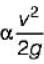+ ρ/y + z (here p is negative).

QUESTION: 3

### The loss of head due to friction in a pipe of uniform diameter in which viscous flow is taking place is where Re = flow Reynold’s number

Solution:
QUESTION: 4

In the Moody diagram the third parameter is ks/D. Here ks is

Solution:
QUESTION: 5

A compound pipe of diameters d1, d2 and d3 having length l1, l2 and l3 respectively, is to be replaced by an equivalent pipe of uniform diameter d and length l. The size of the equivalent pipe is given by

Solution:

For pipes in series equivalent pipe is given by: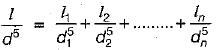For pipes in parallel equivalent pipe is given by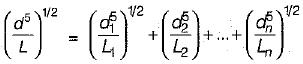QUESTION: 6

In a pipe flow, the shear velocity u. is related to the Darcy-Weisbach friction factor f as u./V =

Solution:

friction factor,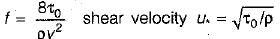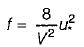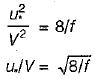QUESTION: 7

Two pipe systems are said to be equivalent when

Solution:
QUESTION: 8

An oil of kinematic viscosity 0.25 stokes flows, through a pipe of diameter 10 cm. The flow is critical., at a velocity of

Solution:

R= 2000 = VD/v
2000 =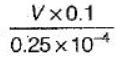⇒ V = 0.5 m/sec

QUESTION: 9

Which one of the following phenomena in a pipe flow is termed as water hammer?

Solution:
QUESTION: 10

The pressure at the summit of a syphon is

Solution:

The centre line of pipe is well above the hydraulic grade line and therefore the pressure is negative or below atmospheric.